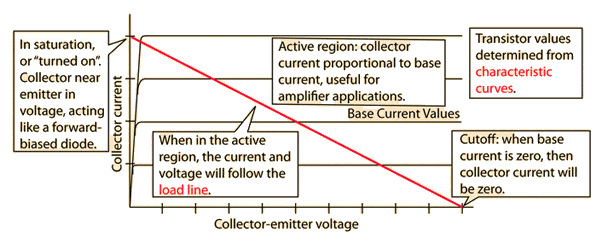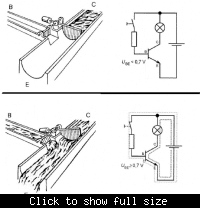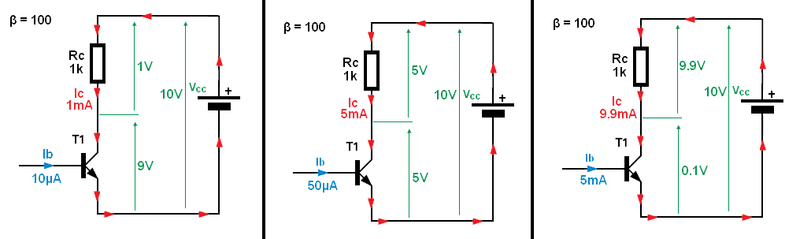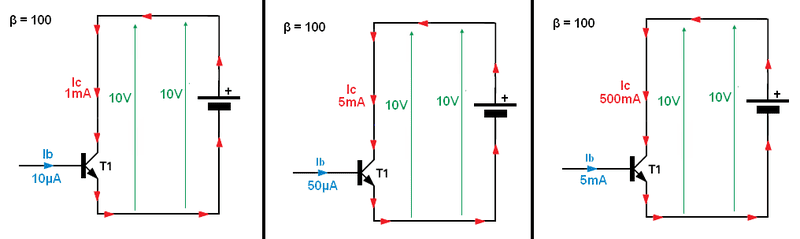Need Help Understanding Transistor Theory: Saturation Region

I'm self learning electronics and trying to wrap my head around its characteristics.

I was looking at this source.

Regarding the active and saturation region graph interpretation on that page, I would like to confirm if my thinking is correct. From that graph, it states that the ratio between i_c and i_b is inconsistent at V_ce < 0.7. Beyond the saturation region, the relationship between i_c and i_b is now constant. We call that beta (β). The breakdown region is when the voltage V_ce reaches (or goes beyond) the voltage V_cc.

From that link: "Suppose VBB and VCE are set to produce a current iB > 0 and VCE=0 V. Then both BE and BC junctions are forward biased. The saturation region corresponds to the case where both junctions are forward biased."
Under what condition would V_ce equal to 0V while in the saturated region besides when i_b = 0 and i_c = 0? Or is it just saying that the starting position of V_ce=0V and it is expected for i_b to increase?

I gathered that the cutoff region occurs beyond the midpoint of 0 volts and where V_ce=V_cc.

I don't understand the concept behind the cutoff region. I would like a clearer explanation on what is the cutoff region. It says the cutoff region is when i_b = 0, but what is i_c? I think I get tripped up over the different values of i_b in that graph and its relationship with the cutoff region, whether those values are considered in the cutoff region or not.

Another question: I was doing a few [URL="http://www.wisc-online.com/Objects/ViewObject.aspx?ID=SSE6104]practice problems[/URL] I found online on transistors where they say to assume that i_c = i_e (or at least, approximately equal). When I was doing those problems I initially made the mistake of calculating i_c by doing i_c = V_cc/R_c, thinking that i_c would equate to the current flowing from V_cc when in actuality, i_c = i_e. I learned that I had to find the current flowing through i_e to get a correct value for i_c. Why is this the case? I would have thought because the base acts as a switch by opening up contact between the collector and the emitter that the current from the emitter, i_e would equal to the current flowing to the collector, i_c based on using V_cc to calculate for i_c using ohm's law.

I also figured that at the linear region of an npn transistor, the emitter-base region goes from forward bias (sat region configuration) to reversed bias: V_Rc + V_c = V_cc. Also, V_Re + V_be = V_b where V_be = 0.7 V. Therefore the voltage drop from the start of the emitter to ground is equal to the voltage V_bb because of forward bias from the base to the emitter.

Thank you everyone.

dlgoff
Gold Member
I don't understand the concept behind the cutoff region. I would like a clearer explanation on what is the cutoff region. It says the cutoff region is when i_b = 0, but what is i_c? I think I get tripped up over the different values of i_b in that graph and its relationship with the cutoff region, whether those values are considered in the cutoff region or not.
Note the red "load line" in this image from http://hyperphysics.phy-astr.gsu.edu/hbase/solids/trans2.html.This link will explain how you use these "characteristic curves" to determine "load lines" for a particular transistor; with numerical examples.

Regards

Thank you for the reply. I understand the cutoff region now. I was trying to find this, but maybe I had been overlooking it: Why is the collector current equal to the emitter current? Why is it not the other way around? Yet the voltage drop from the base to ground is equal to the voltage V_b, while the drop from the source to the end of the collector is equal to the source.

Hi
Your main problem is that you don't understand how BJT work.
But to understand how BJT work, first you need to understand how a constant current source work. Are you familiar with the concept of a current source ?

The BJT's in active region act just like a base current controlled collector current source.
See this example show a BJT in active region.

If base current is flowing the BJT is ON and collector current is BETA (β or Hfe) times larger than the base current (Ic = β*Ib).

And Ic = β *Ib and Ie = Ib + Ic is a basic principle of a transistor "action".

Note that arrow on a BJT (on a emitter) symbol show how current will be flow through BJT. Of course from "+" to "-".

The BJT work very similarly to the tap water valve.
A water valve is always used as a device to control the flow of water. Similarly, always think of a bipolar transistor as a device used to control electric current flow by assistance of a base current.
If base current (Ib) flows hen BJT is ON so there must flow β times Ib current flow through collector. See this pictureBut if we add Rc resistor between Vcc and collector we have this situationAs you can see in first two examples BJT work in active region and Ic current is equal to:
Ic = β*Ib . But in the last example Ic = 100*5mA = 500mA don't holds anymore, do you know why? Because now we have Rc in the circuit so Ohm's and kirchoff's law must hold also. What is the max Ic that can flow in this circuit? We all knows the Ohms law, so Ic_max = Vcc/Rc ≈ 10mA . BJT tries to create a situation in which the collector current Ic = β*Ib = 500mA. So BTJ lowers his collector-emitter voltage to Vcs_sat voltage. The BJT in full ON. Transistor is in saturation region. In saturation Ic = β*Ib don't hold anymore. And in saturation the collector is equal to:
Ic_sat = (Vcc - Vce_sat)/Rc ≈ Vcc/Rc

Here you have a more detailed explanation about saturation region.
I think a reasonably simple yet useful description of the saturation process goes something like this:

Think of a transistor as a device that tries to create a situation in which the collector current, Ic, is β*Ib. The mechanism by which it does this is by controlling the "resistance" of the collector-emitter path through the device. If the present resistance is such that the collector current is less than β*Ib, then the transistor reduces the resistance. This generally results in a lower collector-emitter voltage which means that the voltage dropped across the external components increases which generally allows more collector current to flow. The reverse happens if the present collector current is more than β*Ib in order to reduce it.

But this process can only be carried so far. The transistor can only lower the collector-emitter voltage so far and, once that point is reached, the transistor no longer has any tools in its bag to increase the collector current up to the point it would like it to be, so the collector-emitter voltage stops dropping and the collector current is less β*Ib.

Ideally this saturation voltage would be 0V and the collector-emitter junction would simply look like a short circuit allowing any current to flow as long as it doesn't go above β*Ib (since, at that point, the transistor will come out of saturation and start increasing Vce in order to hold the current to β*Ib).

In practice, real transistors have a saturation voltage that is greater than 0V, but it is generally only a few hundred millivolts.

written by WBahn

Also notice that when base current increases the collector current also increases.
So voltage drop across Rc resistor must also increase (Ohm's law VRc = Ic * Rc).
So V_Rc increases the Vce voltage must drop. Because V_Supply = VRc + Vce.
As you can see when input voltage is increasing ( base current also) the Vce voltage decrease. So we have 180 degrees out of phase.

Attachments

Last edited by a moderator:
Thank you...

Maybe I don't/didn't understand it... (I'll admit that I have trouble wording math concepts properly).

I also found this word document that explains transistors and answered my question as to why when β >> 1 that i_c = i_e. They had explained that i_c = (β/β+1)i_e and as β >> 1, i_c = i_e because that +1 becomes negligible and the equation becomes i_c = (β/β)i_e which can be reduced to 1*i_e or just simply i_e.

Correct me if I'm wrong: When a current source is in a circuit, the current is independent of the voltage if the current source is an independent current source.

If a voltage source and an independent current source are connected, the current through the circuit is equal to the current produced by the current source. The voltage drop is the voltage of the voltage source.

Is it safe to then say that if an independent current source is then placed in a circuit with a resistor and voltage source, the current in that circuit would equal to the current produced by the current source?

I can't see the first picture in your explanation, but I think I understood your explanation.

I also found this word document that explains transistors and answered my question as to why when β >> 1 that i_c = i_e. They had explained that i_c = (β/β+1)i_e and as β >> 1, i_c = i_e because that +1 becomes negligible and the equation becomes i_c = (β/β)i_e which can be reduced to 1*i_e or just simply i_e.

As you should know the emitter current is equal to Ie = Ib + Ic but we also know that a collector current is equal to Ic = β*Ib
So we end-up with this equation for emitter current Ie = Ib + Ic = Ib + β*Ib = (β + 1)*Ib (emitter current is the sum of the base and collector current).
So for example if we have a BJT with a current gain equal to β = 100 and the base current is equal to Ib = 10μA. The collector current will be equal to Ic = 100 * 10μA = 1mA and the emitter current Ie = Ib + Ic = 10μ + 1mA = 1.01mA So we can safely assume base current is negligible and say that Ie ≈ Ic

Correct me if I'm wrong: When a current source is in a circuit, the current is independent of the voltage if the current source is an independent current source.
OK
If a voltage source and an independent current source are connected, the current through the circuit is equal to the current produced by the current source. The voltage drop is the voltage of the voltage source.
OK
Is it safe to then say that if an independent current source is then placed in a circuit with a resistor and voltage source, the current in that circuit would equal to the current produced by the current source?
Yes we can say that.

I can't see the first picture in your explanation, but I think I understood your explanation

Here you have this missing exampleand compare it with this examplesAnd I hope that you can without any problem calculate all current and voltages in this circuit?

Attachments

•1 person